Axial field of a real Helmholtz coil pairThis formula uses the formula for the field due to a finite solenoid to obtain the magnetic field at any point on the axis of a Helmholtz coil pair.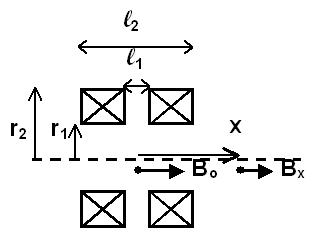Helmholtz coil pair in cross section view.General Case: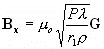where G is the unitless geometry factor: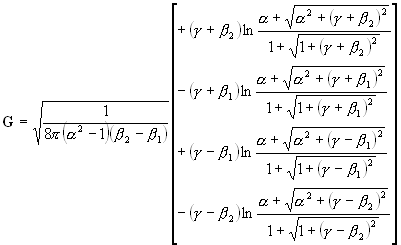Where:,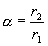,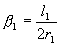,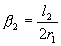Bx is the magnetic field, in teslas, at any point on the axis of the coil pair. The direction of the field is parallel to the coil pair axis.

m o is the permeability constant (1.26x10-6 Tm/A, 1.26x10-4 Tcm/A or 4.95x10-5 Tin/A, for coils measured in meters, centimeters and inches, respectively)

r1 is the inside radius of the coil pair.

r2 is the outside radius of the coil pair.

l1 and l2 are the distances between inner and outer coil faces, respectively.

P is the total power consumed by the coil pair, in watts.

l is equal to (total conductor cross section area)/(total coil cross section area), which ranges from 0.6 to 0.8 in typical coils.

r is the conductor resistivity, in units of ohms-length. The length units must match those of r1.

Note that the units of length may be meters, centimeters or inches (or furlongs, for that matter), as long as the correct value of the permeability constant is used.Special Case: x = 0 The magnetic field measurement point is at the center of the coil pair.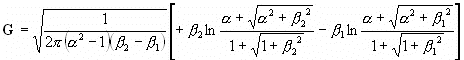Note: for "proper" Helmholtz spacing using coils with a square cross section, the following hold true: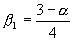and...Magnet Formulas web site, © 2005 by Eric Dennison. I am developing an updated and expanded version of the magnet web site and encourage you to visit this work in progress and comment on what you see. As a self-employed software engineer trying to balance time between work and magnets, your encouragement in building this site is always welcome! Please consider clicking the PayPal link and making a contribution to support the maintenance and development of this site. Thank you for visiting!